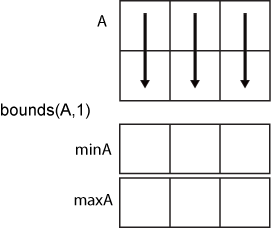# bounds

Minimum and maximum values of an array

## Syntax

``````[minA,maxA] = bounds(A)``````
``[minA,maxA] = bounds(A,"all")``
``````[minA,maxA] = bounds(A,dim)``````
``[minA,maxA] = bounds(A,vecdim)``
``````[minA,maxA] = bounds(___,missingflag)``````

## Description

example

``````[minA,maxA] = bounds(A)``` returns the minimum value `minA` and maximum value `maxA` in an array. `minA` is equivalent to `min(A)` and `maxA` is equivalent to `max(A)`.```

example

````[minA,maxA] = bounds(A,"all")` computes the minimum and maximum values over all elements of `A`.```

example

``````[minA,maxA] = bounds(A,dim)``` operates along the dimension `dim` of `A`. For example, if `A` is a matrix, then `bounds(A,2)` returns column vectors `minA` and `maxA` containing the minimum and maximum values in each row.```

example

````[minA,maxA] = bounds(A,vecdim)` computes the minimum and maximum values based on the dimensions specified in the vector `vecdim`. For example, if `A` is a matrix, then `bounds(A,[1 2])` returns the minimum and maximum values over all elements in `A`, since every element of a matrix is contained in the array slice defined by dimensions 1 and 2.```

example

``````[minA,maxA] = bounds(___,missingflag)``` specifies whether to omit or include missing values in `A` for any of the previous syntaxes. For example, `bounds(A,"missingflag")` includes all missing values when computing the minimum and maximum values. By default, `bounds` omits missing values.```

## Examples

collapse all

Simultaneously compute the minimum and maximum values of a vector.

```A = [2 4 -1 10 6 3 0 -16]; [minA,maxA] = bounds(A)```
```minA = -16 ```
```maxA = 10 ```

Compute the minimum and maximum values in each row of a matrix.

`A = magic(4)`
```A = 4×4 16 2 3 13 5 11 10 8 9 7 6 12 4 14 15 1 ```
`[minA,maxA] = bounds(A,2)`
```minA = 4×1 2 5 6 1 ```
```maxA = 4×1 16 11 12 15 ```

Create a 3-D array and compute the minimum and maximum values in each page of data (rows and columns).

```A(:,:,1) = [2 4; -2 1]; A(:,:,2) = [9 13; -5 7]; A(:,:,3) = [4 4; 8 -3]; [minA1,maxA1] = bounds(A,[1 2]); minA1```
```minA1 = minA1(:,:,1) = -2 minA1(:,:,2) = -5 minA1(:,:,3) = -3 ```
`maxA1`
```maxA1 = maxA1(:,:,1) = 4 maxA1(:,:,2) = 13 maxA1(:,:,3) = 8 ```

To compute the bounds over all dimensions of an array, you can either specify each dimension in the vector dimension argument or use the `"all"` option.

`[minA2,maxA2] = bounds(A,[1 2 3])`
```minA2 = -5 ```
```maxA2 = 13 ```
`[minAall,maxAall] = bounds(A,"all")`
```minAall = -5 ```
```maxAall = 13 ```

Create a matrix containing `NaN` values.

`A = [2 NaN 6 -5; 0 3 NaN 9]`
```A = 2×4 2 NaN 6 -5 0 3 NaN 9 ```

Compute the minimum and maximum values of the matrix, including `NaN` values. For matrix columns that contain any `NaN` value, the minimum and maximum are `NaN`.

`[minA,maxA] = bounds(A,"includenan")`
```minA = 1×4 0 NaN NaN -5 ```
```maxA = 1×4 2 NaN NaN 9 ```

## Input Arguments

collapse all

Input array, specified as a vector, matrix, or multidimensional array.

Data Types: `single` | `double` | `int8` | `int16` | `int32` | `int64` | `uint8` | `uint16` | `uint32` | `uint64` | `logical` | `categorical` | `datetime` | `duration`
Complex Number Support: Yes

Dimension to operate along, specified as a positive integer scalar. If you do not specify the dimension, then the default is the first array dimension of size greater than 1.

Consider an `m`-by-`n` input matrix, `A`:

• `bounds(A,1)` computes the minimum and maximum values in each column of `A` and returns two `1`-by-`n` row vectors.• `bounds(A,2)` computes the minimum and maximum values in each row of `A` and returns two `m`-by-`1` column vectors.Vector of dimensions, specified as a vector of positive integers. Each element represents a dimension of the input array. The lengths of the output in the specified operating dimensions are 1, while the others remain the same.

Consider a 2-by-3-by-3 input array, `A`. Then `[minA,maxA] = bounds(A,[1 2])` returns a 1-by-1-by-3 array for both `minA` and `maxA`. The elements of `minA` and `maxA` are the minimum and maximum values in the corresponding page of `A`, respectively.Missing value condition, specified as one of the values in this table.

ValueInput Data TypeDescription
`"omitmissing"`All supported data typesIgnore missing values in the input array, and compute the minimum maximum over fewer points. If all elements in the operating dimension are missing, then the corresponding elements in `minA` and `maxA` are missing.
`"omitnan"``double`, `single`, `duration`
`"omitnat"``datetime`
`"omitundefined"``categorical`
`"includemissing"`All supported data types

Include missing values in the input array when computing the minimum and maximum. If any element in the operating dimension is missing, then the corresponding elements in `minA` and `maxA` are missing.

`"includenan"``double`, `single`, `duration`
`"includenat"``datetime`
`"includeundefined"``categorical`

## Output Arguments

collapse all

Minimum value, returned as a vector, matrix, or multidimensional array.

Maximum value, specified as a vector, matrix, or multidimensional array.

## Version History

Introduced in R2017a

expand all